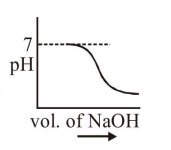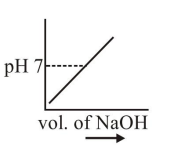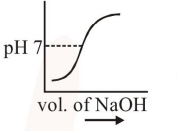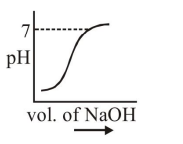# Solve this following

Question:

$100 \mathrm{~mL}$ of $0.1 \mathrm{M} \mathrm{HCl}$ is taken in a beaker and to it $100 \mathrm{~mL}$ of $0.1 \mathrm{M} \mathrm{NaOH}$ is added in steps of $2 \mathrm{~mL}$ and the $\mathrm{pH}$ is continuously measured. Which of the following graphs correctly depicts the change in $\mathrm{pH}$ ?

1.2.3.4.Correct Option: , 3

Solution:

Steep rise in $\mathrm{pH}$ around the equivalence point for titration of strong acid with strong base.